# Quick Search error when link to other table

I have 2 table Propinsi and Kota. I create grid for Kota table where links to Propinsi table. But I found error when running Quick Search. I have tried to find out what makes it error. Do I miss something ? I am sure this is bug.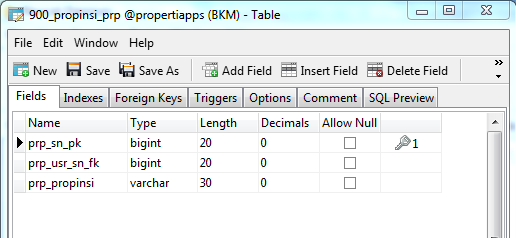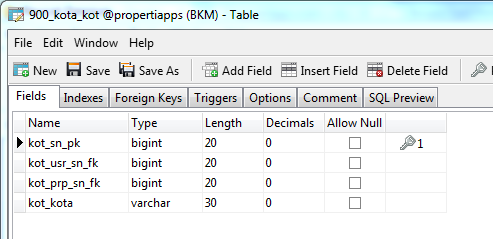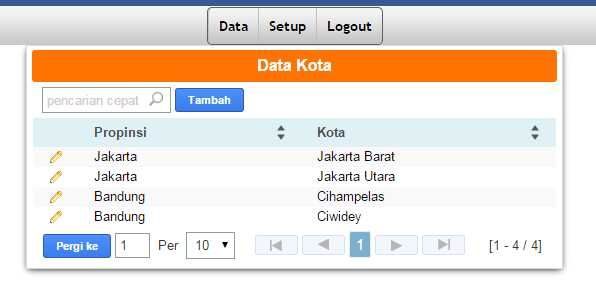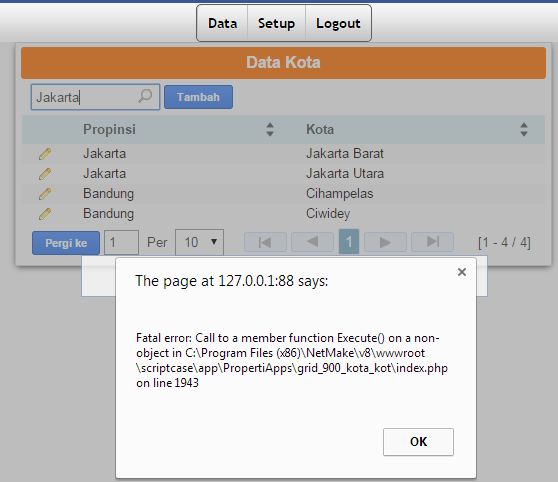Look at lines 1940-1950 at your code post this here …

I don’t write any coding, just default from SC8.

Yes … Post that code and we will see, what is wrong.

This is the code. I took from line 1921 up to 2002, since it is a function.

function SC_lookup_kot_prp_sn_fk(\$condicao, \$campo)
{
\$result = array();
\$nm_comando = “SELECT prp_propinsi, prp_sn_pk FROM 900_propinsi_prp WHERE (prp_propinsi LIKE ‘%\$campo%’)” ;
if (\$condicao == “ii”)
{
\$nm_comando = str_replace(“LIKE ‘%\$campo%’”, “LIKE ‘\$campo%’”, \$nm_comando);
}
if (\$condicao == “df” || \$condicao == “np”)
{
\$nm_comando = str_replace(“LIKE ‘%\$campo%’”, “NOT LIKE ‘%\$campo%’”, \$nm_comando);
}
if (\$condicao == “gt”)
{
\$nm_comando = str_replace(“LIKE ‘%\$campo%’”, “> ‘\$campo’”, \$nm_comando);
}
if (\$condicao == “ge”)
{
\$nm_comando = str_replace(“LIKE ‘%\$campo%’”, “>= ‘\$campo’”, \$nm_comando);
}
if (\$condicao == “lt”)
{
\$nm_comando = str_replace(“LIKE ‘%\$campo%’”, “< ‘\$campo’”, \$nm_comando);
}
if (\$condicao == “le”)
{
\$nm_comando = str_replace(“LIKE ‘%\$campo%’”, “<= ‘\$campo’”, \$nm_comando);
}
\$_SESSION[‘scriptcase’][‘sc_sql_ult_comando’] = \$nm_comando;
\$_SESSION[‘scriptcase’][‘sc_sql_ult_conexao’] = ‘’;
if (\$rx = \$this->Ini->nm_db_conn_PropertiApps->Execute(\$nm_comando))
{
while (!\$rx->EOF)
{
\$chave = \$rx->fields;
\$label = \$rx->fields;
if (\$condicao == “eq” && \$campo == \$label)
{
\$result[] = \$chave;
}
if (\$condicao == “ii” && \$campo == substr(\$label, 0, strlen(\$campo)))
{
\$result[] = \$chave;
}
if (\$condicao == “qp” && strstr(\$label, \$campo))
{
\$result[] = \$chave;
}
if (\$condicao == “np” && !strstr(\$label, \$campo))
{
\$result[] = \$chave;
}
if (\$condicao == “df” && \$campo != \$label)
{
\$result[] = \$chave;
}
if (\$condicao == “gt” && \$label > \$campo )
{
\$result[] = \$chave;
}
if (\$condicao == “ge” && \$label >= \$campo)
{
\$result[] = \$chave;
}
if (\$condicao == “lt” && \$label < \$campo)
{
\$result[] = \$chave;
}
if (\$condicao == “le” && \$label <= \$campo)
{
\$result[] = \$chave;
}
\$rx->MoveNext() ;
}
return \$result;
}
elseif (\$GLOBALS[“NM_ERRO_IBASE”] != 1)
{
\$this->Erro->mensagem(FILE, LINE, “banco”, \$this->Ini->Nm_lang[‘lang_errm_dber’], \$this->Ini->nm_db_conn_PropertiApps->ErrorMsg());
exit;
}
}

Please show the SQL select statements for the grids …

Here it is.

SELECT
kot_sn_pk,
kot_usr_sn_fk,
kot_prp_sn_fk,
kot_kota
FROM
900_kota_kot
WHERE
kot_usr_sn_fk = [usr_sn_pk]

Aparently this: \$this->Ini->nm_db_conn_PropertiApps is not created. Hence the error. It says that the Execute is going wrong since the point that the thing before the execute is not an object where it should be. So your error is before that. Check if the database connection is properly made.

I have set the connection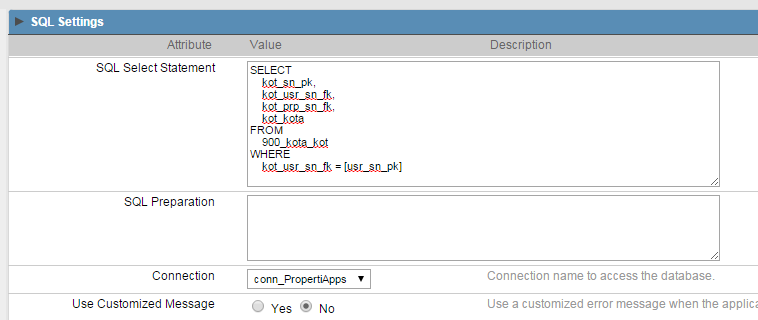Maybe an odd question but did you test your connection? Do you have a seperate program (e.g. mysql workbench or so) wuth which you can verify that your settings are correct?

Connection is no problem since other menus run smoothly.

Connection is no problem since other menus run smoothly.

OK. Solved already. SQL syntax needs to be changed.
Thank you all.

[QUOTE=ali.dewanto;32571]OK. Solved already. SQL syntax needs to be changed.
Thank you all.[/QUOTE]

Hi - can you explain what that SQL syntax issue was? I am getting the same type of error and have not been able to resolve.

Thanks!